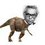# Oh wow!

If $\quad a^3+b^3+c^3 = (a+b+c)^3$ ,

Prove that $\quad \large a^{2n+1}+ b^{2n+1}+ c^{2n+1} = (a+b+c)^{2n+1}$ for all $n \in N$

Nice proofs are always welcome. :)Note by Nihar Mahajan
6 years, 2 months ago

This discussion board is a place to discuss our Daily Challenges and the math and science related to those challenges. Explanations are more than just a solution — they should explain the steps and thinking strategies that you used to obtain the solution. Comments should further the discussion of math and science.

When posting on Brilliant:

• Use the emojis to react to an explanation, whether you're congratulating a job well done , or just really confused .
• Ask specific questions about the challenge or the steps in somebody's explanation. Well-posed questions can add a lot to the discussion, but posting "I don't understand!" doesn't help anyone.
• Try to contribute something new to the discussion, whether it is an extension, generalization or other idea related to the challenge.
• Stay on topic — we're all here to learn more about math and science, not to hear about your favorite get-rich-quick scheme or current world events.

MarkdownAppears as
*italics* or _italics_ italics
**bold** or __bold__ bold
- bulleted- list
• bulleted
• list
1. numbered2. list
1. numbered
2. list
Note: you must add a full line of space before and after lists for them to show up correctly
paragraph 1paragraph 2

paragraph 1

paragraph 2

[example link](https://brilliant.org)example link
> This is a quote
This is a quote
    # I indented these lines
# 4 spaces, and now they show
# up as a code block.

print "hello world"
# I indented these lines
# 4 spaces, and now they show
# up as a code block.

print "hello world"
MathAppears as
Remember to wrap math in $$ ... $$ or $ ... $ to ensure proper formatting.
2 \times 3 $2 \times 3$
2^{34} $2^{34}$
a_{i-1} $a_{i-1}$
\frac{2}{3} $\frac{2}{3}$
\sqrt{2} $\sqrt{2}$
\sum_{i=1}^3 $\sum_{i=1}^3$
\sin \theta $\sin \theta$
\boxed{123} $\boxed{123}$

## Comments

Sort by:

Top Newest

Well, if the first condition is met, then one of these are true, $a+b=0$, $b+c=0$, $c+a=0$, and so the next condition follows immediately.

- 6 years, 2 months ago

Log in to reply

@Michael Mendrin @Calvin Lin Sir , can you extend my approach that I have written above?

- 6 years, 2 months ago

Log in to reply

I can't go anywhere with that approach either. But if I just solve it for $=0$, I get the condition $a+b=0$, $b+c=0$, or $c+a=0$, and so the rest becomes easy.

- 6 years, 2 months ago

Log in to reply

Well I THINK it can be proved without induction.@Nihar Mahajan @Vaibhav Prasad I will write it in short. It can easily be derived that $(a+b)(b+c)(a+c)=0$ Now we have 3 cases in first all the 3 terms are 0 , or any 2terms are 0 or only one term is 0.

Case$(a+b)=0$

$a=-b$

So now just substitute a = -b in the equation (which is to be proved)

That will give $c^{2n+1} = c^{2n+1}$

- 6 years, 2 months ago

Log in to reply

See my approach:

$a^3+b^3+c^3=(a+b+c)^3-3(a+b+c)(ab+bc+ac)+3abc \\ \Rightarrow 3(a+b+c)(ab+bc+ac) = 3abc \\ (a+b+c)\left(\dfrac{ab+bc+ac}{abc}\right)=1 \quad or \quad (a+b+c)\left(\dfrac{1}{a}+\dfrac{1}{b}+\dfrac{1}{c}\right)=1$

Am not able to do more than this...

- 6 years, 2 months ago

Log in to reply

I will try using this method and tell you about it . But this method does not work when a or b or c=0 and if its right then it proves 0/0=1 (lol was it so easy)

- 6 years, 2 months ago

Log in to reply

Yeah , my method has $a,b,c \neq 0$.

- 6 years, 2 months ago

Log in to reply

I will try solving it by your method and tell you tomorrow (well If I can solve it).

- 6 years, 2 months ago

Log in to reply

Take $a+c=x$ Then $(b+x)(bx+ac) = abc$

Multiplying and simplifying gives us $x(b^{2}+bx+ac )= 0$substitute x Then Further factorizing gives us $a+b)(b+c)(a+c) = 0$

Now the method goes as usual (given in my method).

- 6 years, 2 months ago

Log in to reply

@Kalash Verma I understood your method. I am asking , can you extend my approach furthur?

- 6 years, 2 months ago

Log in to reply

@Nihar Mahajan Well actually its the extension of ur approach. In the third line I have replaced a+c by x and then I have explained above.In the end both results in same factors .

- 6 years, 2 months ago

Log in to reply

Thanks for your help! :) @Kalash Verma

- 6 years, 2 months ago

Log in to reply

Don't mention it.AND you have 703 followers and 703 following. Wow.congrats

- 6 years, 2 months ago

Log in to reply

Oh ,,, I got your method... Thanks!

- 6 years, 2 months ago

Log in to reply

Induction on $n$ ??

- 6 years, 2 months ago

Log in to reply

Most probably .. xD

- 6 years, 2 months ago

Log in to reply

I always prefer induction... even though it's kind of a shortcut

- 6 years, 2 months ago

Log in to reply

×

Problem Loading...

Note Loading...

Set Loading...